# 矩形截面通道中颗粒惯性聚集现象的力学成因The Mechanical Causes of Inertial Focus of Particles in Rectangular Section Channel

DOI: 10.12677/MOS.2021.101009, PDF, HTML, XML, 下载: 84  浏览: 153  国家自然科学基金支持

Abstract: Based on the “Galileo Principle of Relativity”, a numerical model is proposed to describe the steady and constant motion of spherical particles, and the spatial distribution of the inertial lift of spherical particles in rectangular cross-section channel is studied by combining with CFD technology. The factors that cause the change of the stable aggregation position are analyzed, which can provide guidance for the study of the inertial aggregation of particles in rectangular cross-section channel and the controlled commercial application. The result of research shows that in the rectangular cross-section channel, the particles are affected by the horizontal component of the inertial lift, moving away from the center of the short side wall and towards the long side wall, so as to make the two stable aggregation positions that exist should disappear. Different Reynolds number of fluid will cause different changes in the vertical component of the inertial lift of particles. The ratio of height to width of the cross-section channel affects the distribution characteristics of horizontal components of inertial lift, and the rotation effect of particles is the main influencing factor of this phenomenon.

1. 引言

Segré & Silberberg于1961年通过实验   研究了悬浮(颗粒与流体的密度相等)颗粒在圆管直通道内随流体运动的情况。研究结果表明：颗粒在聚集过程中表现出自发性，即无需额外施加电或磁场，以现象为基础制作的微流控芯片已成功实现了颗粒和细胞的分离。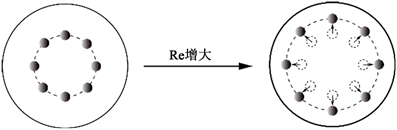(a) 圆形截面通道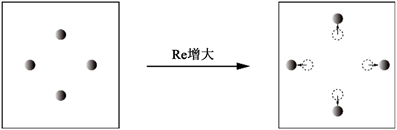(b)方形截面通道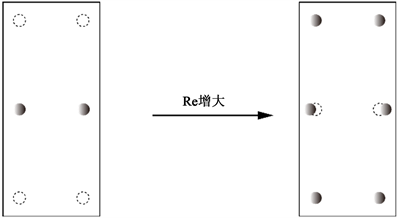(c) 矩形截面通道

Figure 1. The change of particle inertial focuses

Asmolov  利用摄动法从理论上获得颗粒在微通道内的力学特性，然而使摄动解成立的前提是基于“点颗粒”假设，颗粒的存在对其周围流场无影响。但实际情况中并不能忽略颗粒的大小。Carlo et al.  基于“相对运动模型”，数值研究了方形截面管道流动中颗粒的横向升力，通过数据拟合的方法获得了该升力各个部分的近似表达式；王企鲲等  数值研究了颗粒在微通道中的力学特性，但是在计算中忽略颗粒自身旋转。

2. 计算模型与方法

2.1. 数值计算模型简介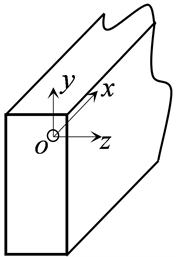(a) 相对模型示意图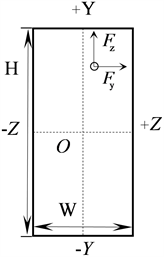(b) 通道截面示意图

Figure 2. Relative motion model

${F}_{L}={∯}_{\Sigma }n\cdot P\text{d}S$ (1)

${F}_{y}=j\cdot {F}_{L}$ (2)

${F}_{z}=k\cdot {F}_{L}$ (3)

2.2. 数值计算方法

$\left\{\begin{array}{l}\nabla \cdot W=0\\ \left(W\cdot \nabla \right)W=-\frac{1}{\rho }\nabla p+\upsilon {\nabla }^{2}W\end{array}$ (4)

$V=W+{U}_{p}$ (5)

$S=\frac{1}{2}\left[\left(\nabla W\right)+{\left(\nabla W\right)}^{\text{T}}\right]$ (6)

$P=-pI+2\mu S$ (7)

2.3. 相关物理参数

${C}_{Fy}=\frac{{F}_{y}}{\rho {U}^{2}{a}^{4}/{D}^{2}}$ (8)

${C}_{Fz}=\frac{{F}_{z}}{\rho {U}^{2}{a}^{4}/{D}^{2}}$ (9)

${a}^{+}=\frac{a}{D}$ (10)

${y}^{+}=\frac{Y}{H/2}$ (11)

${z}^{+}=\frac{Z}{W/2}$ (12)

$Re=\frac{\rho UD}{\mu }$ (13)

$RA=\frac{H}{W}$ (14)

3. 结果与分析

3.1. 短边中线上惯性聚集点消失的力学分析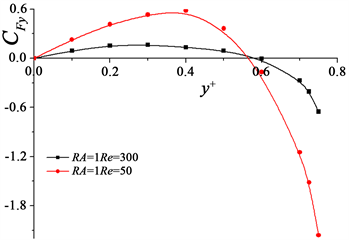(a) RA = 1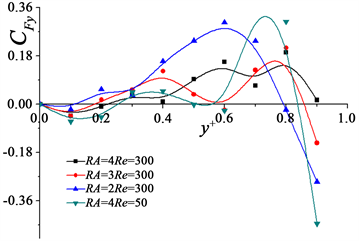(b) RA > 1

Figure 3. The distribution of the Fyof particle on the short side of the middle lineFigure 4. The comparison of Fz of particles in different directions on the short side middle line

3.2. 颗粒惯性聚集形态的力学分析Figure 5. The CFZ of the particle on the lateral position in different y+ positionsFigure 6. The CFy of particle at different positions near the long side wall

3.3. 惯性升力水平分量差异性分布成因分析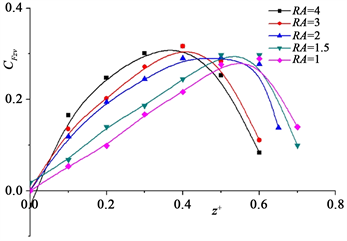(a) CFZW的分布情况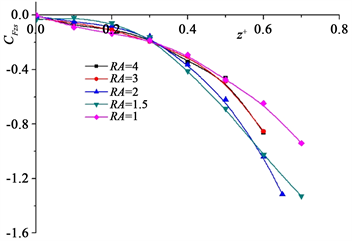(b) CFZS的分布情况

Figure 7. The distribution of different parts of Fz on the particle

4. 结论

1) 矩形截面通道中，在不可忽视的惯性升力水平分量Fz的作用下，颗粒仅于长壁附近存在稳定聚集点，在短壁中心附近已不存在稳定聚集位置。

2) 矩形截面通道中，流体雷诺数会影响惯性升力竖直分量Fy的分布特征。当Re较小时，颗粒只会在长边壁面中线上的特定位置，形成两个稳定的惯性聚集点；当Re增大时，原本仅存在与长边壁面中心处的两个惯性聚集点增加为六个，通道截面的四个直角区域也出现了颗粒的惯性聚集点。

3) 对比方形和矩形截面通道，高宽比RA会影响惯性升力水平分量Fz的分布特征。当通道高宽比RA > 1时，惯性升力水平分量Fz的分布特征会发生突变，之后随着高宽比RA的增大，颗粒的惯性聚集位置向长边壁面方向移动；高宽比RA对于惯性升力水平分量Fz的影响，主要是通过颗粒的自转效应实现的。

  Di, C.D. (2009) Inertial Microfluidics. Lab on A Chip, 9, 3038-3046. https://doi.org/10.1039/b912547g  Serge, G. and Silberberg, A. (1961) Radial Particle Displacements in Poiseuille Flow of Suspension. Nature, 189, 209-210. https://doi.org/10.1038/189209a0  Zhang, J., Yan, S., Yuan, D., et al. (2015) Fundamentals and Applications of Inertial Microfluidics: A Review. Lab on A Chip, 16, 10-34. https://doi.org/10.1039/C5LC01159K  Matas, J.P., Morris, J.F. and Guazzelli, É. (2003) Inertial Migration of Rigid Spherical Particles in Poiseuille Flow. Journal of Fluid Mechanics, 515, 171-195. https://doi.org/10.1017/S0022112004000254  Choi, Y.S., Seo, K.W. and Lee, S.J. (2011) Lateral and Cross-Lateral Focusing of Spherical Particles in a Square Microchannel. Lab on A Chip, 11, 460-465. https://doi.org/10.1039/C0LC00212G  Zhou, J. and Papautsky, I. (2013) Fundamentals of Inertial Focusing in Microchannels. Lab on A Chip, 13, 1121-1132. https://doi.org/10.1039/c2lc41248a  Kim, Y.W. and Yoo, J.Y. (2008) The Lateral Migration of Neutral-ly-Buoyant Spheres Transported through Square Microchannels. Journal of Micromechanics & Microengineering, 18, 065015. https://doi.org/10.1088/0960-1317/18/6/065015  王企鲲. 管流中颗粒“惯性聚集”现象的研究展及其在微流动中的应用[J]. 力学进展, 2012, 42(6): 692-703.  Asmolov, E.S. (1999) The Inertial Lift on a Spherical Particle in a Plane Poiseuille Flow at Large Channel Reynolds Number. Journal of Fluid Mechanics, 381, 63-87. https://doi.org/10.1017/S0022112098003474  Carlo, D.D., Edd, J.F., Humphry, K.J., et al. (2009) Particle Segregation and Dynamics in Confined Flows. Physical Review Letters, 102, 094503. https://doi.org/10.1103/PhysRevLett.102.094503  王企鲲. 微通道中弹性颗粒所受惯性升力特性的数值研究[J]. 机械工程学报, 2015, 51(14): 160-166.  倪晋仁. 固液两相流基本理论及其最新应用[M]. 北京: 科学出版社, 1991.